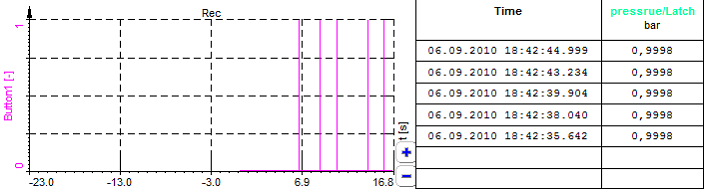# Operators and mathematical function

Dewesoft beside General mathematics in Basics operators allows different types of functions, which are grouped in six tabs in Other math functions section of Formula editor:

Dewesoft supports array channels -> see -> Arrays.

## Basics operators

Dewesoft offers following general mathematical functions - Basic operators section:

General mathematics

Function Syntax Description
+ expression1 + expression2 expression1 plus expression2
- expression1 - expression2 expression1 minus expression2
× expression1 * expression2 multiplies expression1 by expression2
/ expression1 / expression2 divides expression1 by expression2
( ) (expression) brackets expression
^ expression1 ^ expression2 raises expression1 to the power of expression2
DIV expression1 DIV expression2 integer part of division of expression1 by expression2
MOD expression1 MOD expression2 rest of a division of expression1 by expression2

DIV integer division (Delivers the integer part of the division)

• 420 DIV 720 = 0
• 740 DIV 720 = 1

MOD (Delivers the rest of a division) MOD is calculated using the following equation:

$$a\ MOD\ b = a - b * trunc\left(\frac{a}{b}\right)$$ where trunc is the truncate to integer function.

Examples:

• 420 MOD 720 = 420
• 740 MOD 720 = 20
• -8 MOD 3 = -2
• 8 MOD -3 = 2

## Mathematical functions types

All functions have a short hint. When we move the mouse over the icons, we get an explanation of the function and its syntax.

### Functions

The Functions tab contains additional mathematical functions.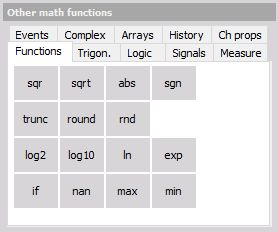Function Syntax Description
SQR SQR(expression) square
SQRT SQRT(expression) square root
ABS ABS(expression) absolute value of expression
SGN SGN(expression) sign, expression > 0: output = 1, expression = 0: output = 0, expression \< 0: output = -1
TRUNC TRUNC(expression) truncate to integer expression
ROUND ROUND(expression) round expression to nearest whole number
RND RND random number between 0 and 1
LOG2 LOG2(expression) logarithm (base 2)
LOG10 LOG10(expression) logarithm (base 10)
LN LN(expression) natural logarithm
EXP EXP(expression) exponent (expression)
IF IF(expression) Create IF sentence
NAN not a number
MAX MAX(expression) calculates the max value of expression
MIN MIN(expresion) calculates the min value of expression

Functions examples and their explanations:

SQR - square sqr(4)= 16, sqr(-3)= 9 The square of a number is the number multiplied with itself. No matter if the number is pos. or neg. the result will be always positive.
SQRT - square root sqrt(16) = 4, sqrt(9) = 3 The square root of number (inverse function of SQR)

INFO: SQRT of a negative number sqrt(-9) will always deliver 0 instead of a complex number in DeweSoft.

ABS - absolute value ABS(45.34) = 45.34, ABS(-33.12) = 33.12, ABS(0) = 0 Calculates the absolute value of number or a channel.
SGN - Sign sgn(-8.124) = -1, sgn(19.345) = 1, sgn(0) = 0 Sign function delivers 0 if input channel or number is 0.
TRUNC - truncate function Trunc(1452.457) = 1452, Trunc(-1452.457) = -1452 Converts a number into integer. So every number or channel which is converted with the Trunc function will loose the part after the comma. It will become an integer value.It is not rounded so either: Trunc(86.248) = 86 and also Trunc(86.848) will give 86

Example: Separate CAN GPS Signal to DEG:MIN,xxx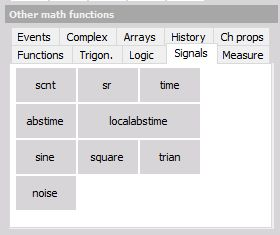The VGPS Longitude and Latitude signals received over the CAN Bus should be separated into DEG:MIN,xxx. The CAN data is received in MIN like it is shown below. With math functions, the regular GPS display should be produced. Below the result is shown.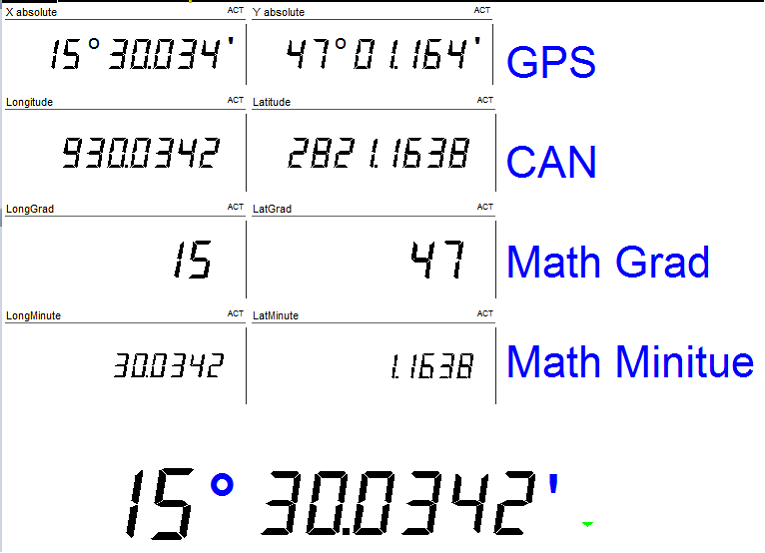MATH: trunc(‘Latitude’/60) dividing by 60 will convert it from minutes to deg, where the trunk function will eliminate the digits after the comma. -> result [°].

((‘Latitude’*100000) mod 6000000) / 100000  will provide the minutes and the rest.

Because the MOD function only delivers the rest in integer we have to multiply the latitude with 100000 and Use mod with 60 *100 000 = 6000 000 and divide the result again with 100 000 to get the result.

ROUND - round function round(14.43) = 14, round(14.501) = 15, round(-14.492) = -14, round(-14.51) = -15 Rounds a number or channel depending on the digits after the comma to an integer value. 3.xxx if xxx is bigger than or equals 0,5 it will be rounded up to the next integer value.

HINT: If you want to round 14.43 to 10, or 136.3724 to 140 simply divide the value or channel by 10 round it and multiply the result by 10.

round(13.63724)*10 = 140

If you want to round 136.3724 to 136.4 multiply the value or channel with 10, round it, and divide it by 10.

round (1363.724)/10 = 136.4

RND - random RDN Creates random numbers with the selected sampling rate between 0 and 1. So if a sample rate of 1000Hz is selected, 1000 values per second are created.
LOG2 - Logarithm base 2 Log2(8) = 3, Log2(a) = b Calculates the logarithm ( base 2) of a number or an input channel. The logarithm extracts b from an equation 2b = a.
LOG10 - Logarithm base 10 Log10(100) = 2, Log2(a) = b Calculates the logarithm (base 10) of a number or an input channel. The logarithm extracts b from an equation 10b = a.
LN- Natural logarithm base e LN(100) = 2, LN(a) = b Calculates the natural logarithm (base e=2.71828…) of a number or an input channel. The logarithm extracts b from an equation 2.71828b = a.
EXP - exponent function of e EXP(1) = 2.71828, EXP(b) = a Calculates the exponential function of e from a number or an input channel.The logarithm extracts b from an equation 2.71828b = a
IF/NAN - If sentence, not a number function IF(condition,result1,result2) Outputs either the result1 or result2 depending on condition. condition: Expects a true false input.

EXAMPLE: (Channel >= 12)

So if the channel, could be a number or any Dewesoft channel, is equal or bigger than 12, the Condition becomes true and result1 will become rue. Otherwise, the condition is false and result will become true.

Example: IF(‘ID’>=1,’velocity’,’displacement’)

If the ID channel is equal or bigger than 1, the statement is true and the velocity channel will be chosen as output, otherwise displacement. So either velocity or displacement is used for output depending on the condition, both at the same time are not possible.

NAN(not a number)

Example: if(‘STWH_ANGLE’>=90,’velocity’,NAN)

‘STWH_ANGLE’ = CAN Channel (asynchronous)

‘Velocity’ = analogue input channel (synchronous)

The channel will deliver only a NAN if STWH_Angle is smaller than 90deg. And if the time base of the math channel is forced to asynchronous output. Otherwise because of a sync. channel is used in the formula (velocity) the formula will output a Sync. channel and NAN will become zero.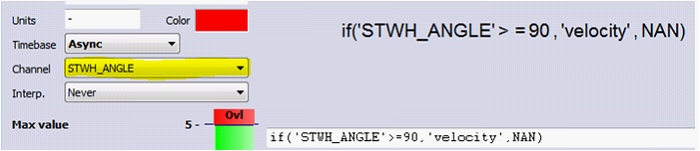The picture above is showing the Timebase setting of the math channel. The async. channel has to be used in the formula otherwise it is not possible to select it in the channel selector. The interpolation has to be set to never.

MAX - Maximum function of more channels MAX(Channel1,Channel2) Checks both channels and outputs the maximum value of one of the channels.

Example: max(‘pressure1’,’pressure2’)

pressure1 and pressure2 are two analogue input channels.

pressure1 3 4 6 8
pressure2 2 5 4 7
output 3 5 6 8

The higher value if both channels will be output.

NOTE: Also, multiple max could be used in one formula.

max(max(‘pressure1’,’pressure2’),’pressure3’)

### Trigonometry

The Trigonometry tab contains all required angle based functions.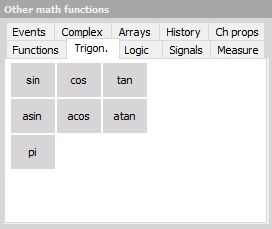Function Syntax Description
SIN SIN(expression) sine of expression [radian]
COS COS(expression) cosine of expression [radian]
TAN TAN(expression) tangent of expression [radian]
PI PI constant piPI
ASIN ASIN(expression) arc-sine of expression
ACOS ACOS(expression) arc-cosine of expression
ATAN ATAN(expression) or ATAN(x,y)arc-cosine of expression arc-tangent of expression or arc-tangent of x/y

### Logic

The Logic tab contains required logic functions. Please be aware that the logic operators OR, AND, NOT and XOR works only with PURE digital values - 0 and 1. Therefore all analog channel must be first digitized before used on this operators (like ‘velocity’<100).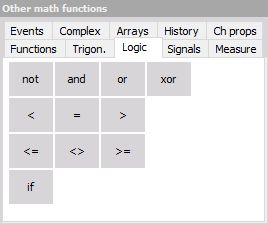Function Syntax Description
\< expression1 \< expression2 if expression1 is less than expression2 then output is 1, else 0
\= expression1 = expression2 if expression1 is equal to expression2 then output is 1, else 0
> expression1 > expression2 if expression1 is greater than expression2 then output is 1, else 0
\<= expression1 \<= expression2 if expression1 is less than or equal to expression2 then output is 1, else 0
\<> expression1 \<> expression2 if expression1 is less or greater than expression2 then output is 1, else 0
>= expression1 >= expression2 if expression1 is greater than or equal to expression2 then output is 1, else 0
NOT NOT expression negation; expression = 0: 1; expression = 1: 0;
AND expression1 AND expression2 logic and, 1 AND 1 = 1, 1 AND 0 = 0, 0 AND 1 = 0, 0 AND 0 = 0
OR expression1 OR expression2 logic or, 1 OR 1 = 1, 1 OR 0 = 1, 0 OR 1 = 1, 0 OR 0 = 0
XOR expression1 XOR expression2 logic exclusive or, 1 XOR 1 = 0, 1 XOR 0 = 1, 0 XOR 1 = 1, 0 XOR 0 = 0

### Signals

Depending on the application, generated signals may be required. Therefore you can select the common signal forms in the Signals tab.Signal functions and generation:

Function Syntax Description
SCNT SCNT Number of samples acquired
SR SR Sample rate of acquisition
TIME TIME Time elapsed (in seconds)
SINE SINE(f [,ps]) Generates a sine wave with frequency f [Hz] optionally with phase shift ps [radian]
SQUARE SQUARE(f [,ps ]) Generates a square wave with frequency f [Hz] optionally with phase shift ps [radian]
TRIAN TRIAN(f [,ps]) Generates a triangle wave with frequency f [Hz] optionally with phase shift ps [radian]
NOISE NOISE Generates noise (random number between ±1)

Signals examples and their explanations:

Sample counter - Delivers the samples acquired from start of the measurement. The counter will be rest at start storing.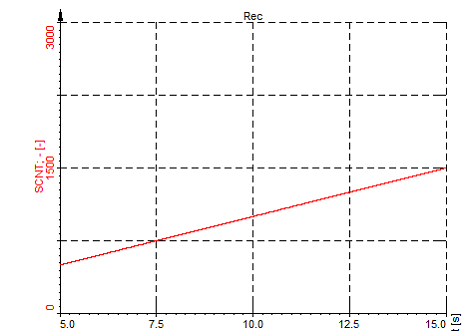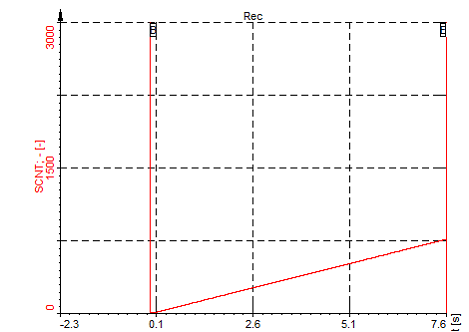The above picture is showing the result, while at the bellow picture the reset after start storing could be seen.

Create Angle Signal

EXAMPLE: If external clocking is used and the signal should be shown in an XY diagram angle based, together with the MOD function we can create an angle signal.

Let’s assume we are using an encoder with 720pulses/rev.

SCNT MOD 720 will deliver a sawtooth which runs from 0 to 720. To get the angle we have to multiply it by 0,5deg so at the end we get this formula.

SCNT MOD 720 * 0.5 this channel could be used in an XY diagram to show the resulting angle based.

The only disadvantage will be, if we get the wrong pulses from the encoder (noise, spikes), the angle signal will shift.

If a TRG pulse is also available, CLK and TRG, this signals could be also routed to a Counter, where this is eliminated.

Because the TRG PULS will reset the counter every revolution.

TIME Time function Is providing the elapsed time of the measurement in seconds.

This is a similar function to SCNT. The only difference is, instead of samples we get the time in seconds, independent which sampling rate is used.

Also, the TIME function is reset at start storing.

The resolution is linked with the sampling rate.

The screenshot below is showing the time function in a recorder. We could see that the resolution is 0,01s and also dt=10ms which results in a sampling rate of 100Hz. So the time will count up 0,01s after every sample at sampling rate of 100Hz or 0,001s at sampling rate 1kHz.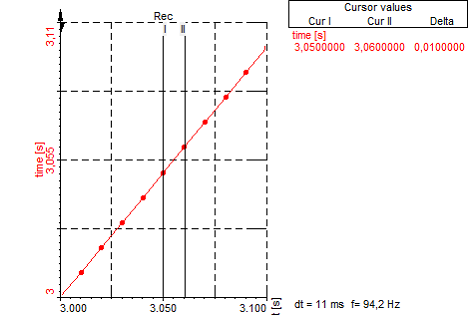EXAMPLE: Show actual value averaged every 10s in a list and export it to Excel.

MATH: (Time MOD 60)

This will create a sawtooth with a period time of 60s. Look at the picture below. This is channel will be used as an event channel in the LATCH math to average the actual channel and show it in a list. The averaged values could be exported to Excel or TXT asynchronously. To export it asynchronously at Export only the asynchronous channels have to be selected.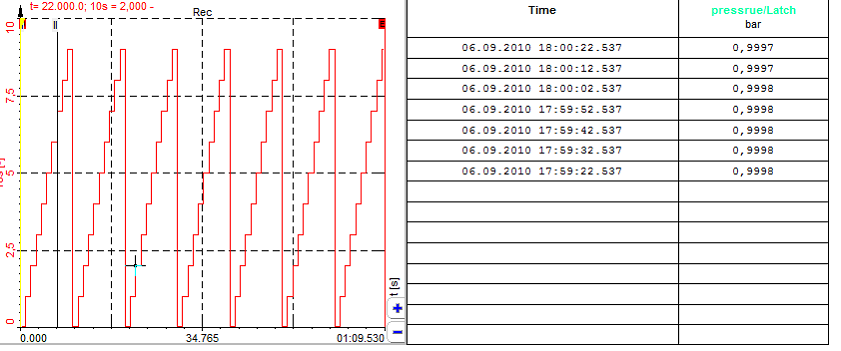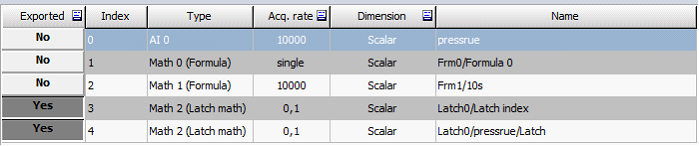NOTE: Generated signals can only be used for mathematics, display and storage. It is not possible to use them for analog output!

If you require analog output (e.g. from a function generator) you have to use the DEWESOFT-OPT-FG option.

For details about Function generator see -> User Guide -> Function Generator.

### Measure

Different measurement values and information are available in the Measure tab. Again here the conditions have to be logic operators. It will again not work to write ECNT(‘Input TTL’) and assume that the transition will be done on value bigger than 1. The correct equation would be ECNT(‘Input TTL’>2.5) where
‘Input TTL’>2.5 give logical 0 or 1.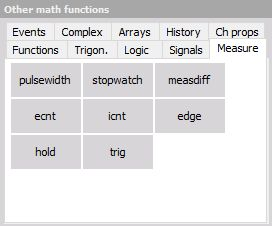for examples see: -> Example of measure functions -> Example of stopwatch

PULSEWIDTH PULSEWIDTH(cond [, rearm ]) Measures time [s] between two cond edges (cond jumps from 0 to 1), rearm edge optional
STOPWATCH STOPWATCH(cond1, cond2 ) Measures time [s] between cond1 and cond2 edges (cond jumps from 0 to 1)
MEASDIFF MEASDIFF(value, cond1, cond2) Measures value difference between cond1 and cond2 edges (cond jumps from 0 to 1)
EDGE EDGE(cond [, rearm ]) Returns 1 when the cond changes from 0 to 1 and have again optional rearm conditions
ECNT ECNT(cond ) Counts number of edges for cond (cond jumps from 0 to 1)
ICNT ICNT(cond ) Counts all the samples where the cond have a logical value 1
HOLD HOLD(value, cond [, rearm ]) shows and holds value, when cond goes from 0 to 1 with optional reams condition
TRIG TRIG has a value of 1 when a store condition appears

Measure examples and their explanations:

HOLD - Hold function

Hold(value,latch condition,[rearm condition])

The hold function is used to latch or hold a single value if a condition is met.

EXAMPLE 1: **Hold(‘pressure’, ‘Temperature’> 30)

Hold(value,latch condition)**

In the example above the function will hold the actual pressure if the temperature is higher or equal 45deg.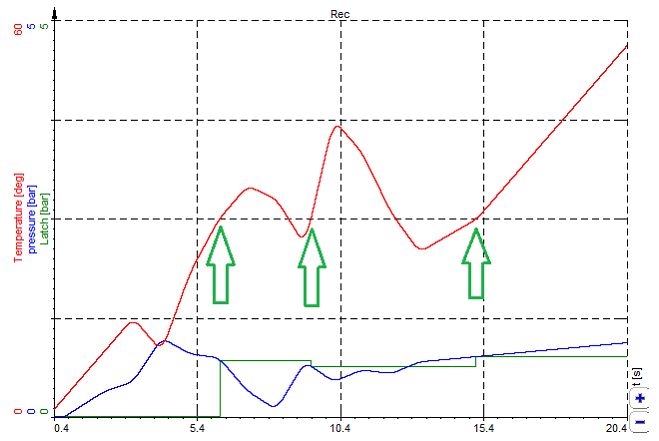The picture above is showing the function in a recorder. All the time when the temperature exceeds 30deg (green arrows), the actual pressure channel is latched.

EXAMPLE 2: Hold(‘pressure’, ‘Temperature’> 30, ‘Temperature’< 28) Hold(value, latchcondition, [rearmcondition])

The function could be extended with an ‘rearmcondition’. After the ‘latchcondition’ occurred, the ‘rearmcondition’ has to be met first before a new ‘latchcondition’ and therefore a new latch could occur. This is used to filer the ‘latchcondition’ a little bit. Imagine the ‘latchcondition’ channel has noise on it or is fluctuating around the level (30deg +-0,2deg) which would cause an unintentional LATCH.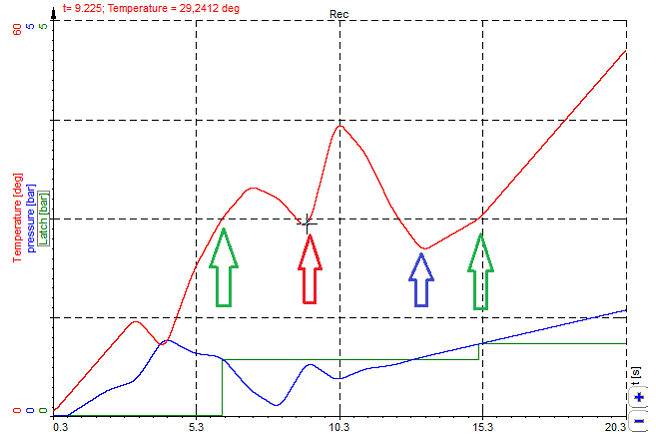The picture above will illustrate this function:

1. Temperature rises above 30 deg -> LATCH 1 - green arrow

2. Temperature goes below 30deg and back above 30 deg -> no LATCH because the temperature did no go under       28deg, therefore, the LATCH did not occur. - red arrow.

3. Temperature goes below 28 deg -> rearm condition is complied - blue arrow

4. Temperature exceeds again 30deg -> LATCH 1 is performed again - green arrow

EXAMPLE 3: Remove offset from a Channel.

HOLD(channel, condition)  … will latch the actual value of the channel if condition become true.

MATH: ‘pressure’-hold(‘pressure’,keypressed(49)>0.5)

The actual pressure channel is subtracted with the value latched in the hold function. The hold function will latch the actual pressure if the second statement ‘keypressed(49)>0.5’ become true.

So even during the measurement, an offset compensation could be done by pressing a specific key. The picture below is showing an example. The ‘keypressed(49)’ channel is indicating the pressed key in an additional math channel to make it more obvious.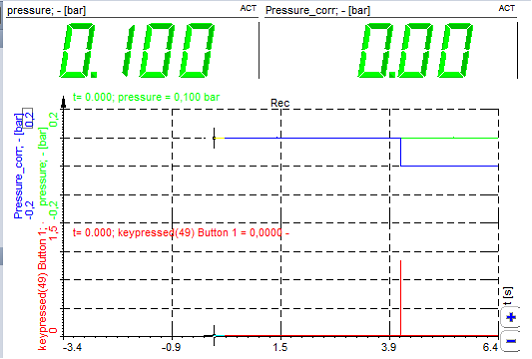### Events

The Events tab allows defining keys as signals. Each time when you press the defined key, you receive a peak in the signal. You can display that e.g. in the recorder, scope, etc.

for examples see -> Example of input events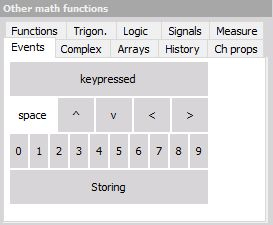Function Syntax Description
KEYPRESSED KEYPRESSED(ASCII code) Generates pulse (transition 0 to 1 and back) if key with matching ASCII code is pressed.

EXAMPLE: KEYPRESSED(32) outputs pulse if Space key is pressed.

Events examples and their explanations:

keypressed(49) - produces a signal from 0 to 1 with a duration of 1 sample if  button is pressed.

A few predefined keys are already prepared as shown in the picture below. So if you press the  button below the line ‘keypressed(49)’ will be automatically added to your formula.Every time the [KEY 1] is pressed on your keyboard a signal is produced.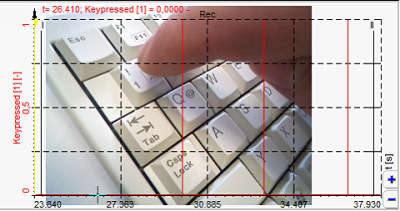Almost any key could be used in the ‘keypressed’ function. The value in the brackets is reflecting the virtual key code in decimal.

Below you will find a list of the most popular keys. You have to convert them from Hex to decimal. So [Key 1] ->31hex -> 49dec. The decimal value has to be entered Into the keypressed function -> keypressed(49).

EXAMPLE: Latch Value into List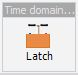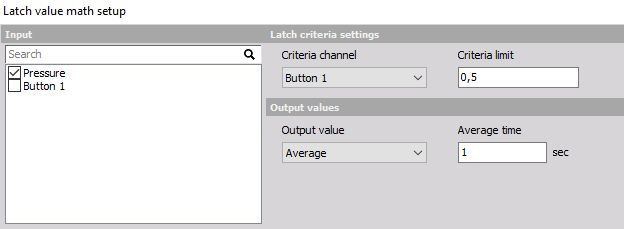MATH: keypressed(49) produces signal from 0 to 1 with a duration of 1 sample if  Button is pressed.

This could be used in the latch Math to latch the actual or average value of an other channel(s) in a list.